Test: Basic Concepts

# Test: Basic Concepts

Test Description

## 20 Questions MCQ Test RRB JE for Electrical Engineering | Test: Basic Concepts

Test: Basic Concepts for Railways 2022 is part of RRB JE for Electrical Engineering preparation. The Test: Basic Concepts questions and answers have been prepared according to the Railways exam syllabus.The Test: Basic Concepts MCQs are made for Railways 2022 Exam. Find important definitions, questions, notes, meanings, examples, exercises, MCQs and online tests for Test: Basic Concepts below.
Solutions of Test: Basic Concepts questions in English are available as part of our RRB JE for Electrical Engineering for Railways & Test: Basic Concepts solutions in Hindi for RRB JE for Electrical Engineering course. Download more important topics, notes, lectures and mock test series for Railways Exam by signing up for free. Attempt Test: Basic Concepts | 20 questions in 60 minutes | Mock test for Railways preparation | Free important questions MCQ to study RRB JE for Electrical Engineering for Railways Exam | Download free PDF with solutions
 1 Crore+ students have signed up on EduRev. Have you?
Test: Basic Concepts - Question 1

### If 120 C of charge passes through an electric conductor in 60 sec, the current in the conductor is:

Detailed Solution for Test: Basic Concepts - Question 1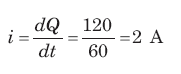Test: Basic Concepts - Question 2

### A solid copper sphere, 10 cm in diameter is deprived of 1020 electrons by a charging scheme. The charge on the sphere is:

Detailed Solution for Test: Basic Concepts - Question 2
• n = 1020
• Q = ne = e * 1020
= -16.02 C
• Charge on sphere will be positive as this electric charge is removed.
Test: Basic Concepts - Question 3

### A lightning bolt carrying 15,000 A lasts for 100 μs. If the lightning strikes an airplane flying at 2 km, the charge deposited on the plane is:

Detailed Solution for Test: Basic Concepts - Question 3

ΔQ = i x Δ t = 15000 x 100μ = 1.5 C

Test: Basic Concepts - Question 4

The energy required to move 120 coulombs through 3 V is:

Detailed Solution for Test: Basic Concepts - Question 4
• W = QV
• W = 120 * 3 = 360 J
Test: Basic Concepts - Question 5

Find the value of i.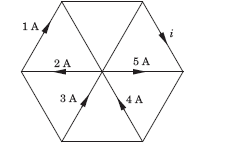Detailed Solution for Test: Basic Concepts - Question 5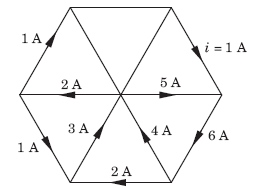• Based on kirchoffs current law, we know that incoming currents at a node is equals to outgoing currents.
• So, based on this 4 and 3 will get canceled with 5 and 2.
• So, finally i = 1.
Test: Basic Concepts - Question 6

In the circuit given, a charge of 600 C is delivered to the 100 V source in a 1 minute. The value of v1 must be: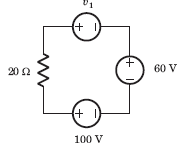Detailed Solution for Test: Basic Concepts - Question 6
• In order for 600 C charge to be delivered to the 100 V source, the current must be anticlockwise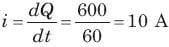• Applying KVL we get:
v1 + 60 - 100 = 10 x 20
v1 = 240 V
Test: Basic Concepts - Question 7

In the circuit of the fig the value of the voltage source E is: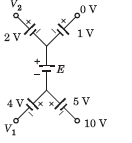Detailed Solution for Test: Basic Concepts - Question 7

Going from 10 V to 0 V: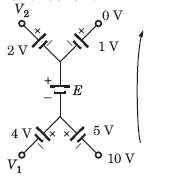• 10 + 5 + E + 1 = 0
• E = -16V
• Minus sign indicates that the polarity of battery should be reversed.
Test: Basic Concepts - Question 8

Consider the circuit graph shown in fig. Each branch of circuit graph represent a circuit element. The value of voltage v1 is: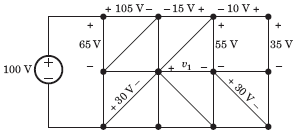Detailed Solution for Test: Basic Concepts - Question 8
• 100 = 65 + v2
⇒ v2 = 35 V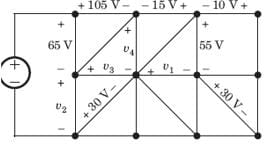• v3 - 30 = v2
⇒ v3 = 65 V
• 105 + v4 - v- 65 = 0
⇒ v4 = 25 V
• v4 + 15 - 55 + v1 = 0
⇒ v1 = 15 V
Test: Basic Concepts - Question 9

What quantity of charge must be delivered by a battery with a Potential Difference of 110 V to do 660J of Work?

Detailed Solution for Test: Basic Concepts - Question 9

► V = W / Q
► Q = W / V = 660 / 110 = 6 C

Test: Basic Concepts - Question 10

Find the value of R1.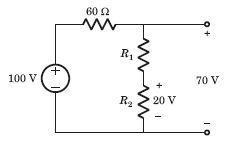Detailed Solution for Test: Basic Concepts - Question 10
• Voltage across 60 Ω resistor = 30 V
• Current = 30 / 60 = 0.5 A
• Voltage across R1 is = (70 - 20) V = 50 V
• R1 = 50 / 0.5 = 100 Ω
Test: Basic Concepts - Question 11

Twelve 6 Ω resistor are used as edge to form a cube.The resistance between two diagonally opposite corner of the cube is

Detailed Solution for Test: Basic Concepts - Question 11

The current i will be distributed in the cube branches symmetrically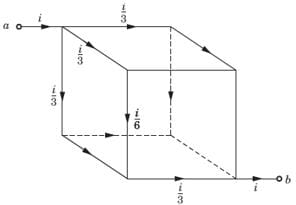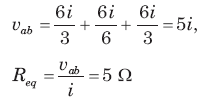Test: Basic Concepts - Question 12

Find the value of v1.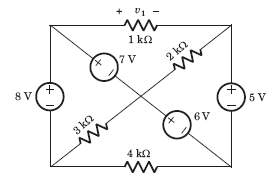Detailed Solution for Test: Basic Concepts - Question 12

If we go from +ve side of 1 kΩ through 7 V, 6 V and 5 V
We get, v1 = 7 + 6 - 5 = 8 V

Test: Basic Concepts - Question 13

The voltage vo in fig is always equal to: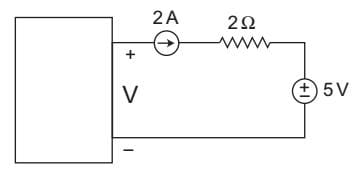Detailed Solution for Test: Basic Concepts - Question 13

From above figure
V – 4 – 5 = 0
or
V = 9 V
Hence alternative (D) is the correct choice.

Test: Basic Concepts - Question 14

In a AC circuit, resistive and total impedance are 10 and 20 ohms respectively. What is the phase angle difference between the voltage and current?

Detailed Solution for Test: Basic Concepts - Question 14
• Cosɸ = R/Z; where R= 10 ohms and Z = 20 ohms
• Cosɸ = 1/2 means phase angle is 60 degree.
Test: Basic Concepts - Question 15

The quantity of a charge that will be transferred by a current flow of 10 A over 1 hour period is _________ ?

Detailed Solution for Test: Basic Concepts - Question 15

We know that,
I=Q / t or Q = I x t = 10 x 1 hours
{since unit of current is Cs-1, therefore time should be in seconds}
∴ Q = 10 x 60 x 60
= 36000 C
= 3.6 x 10C

Test: Basic Concepts - Question 16

A capacitor is charged by a constant current of 2 mA and results in a voltage increase of 12 V in a 10 sec interval. The value of capacitance is:

Detailed Solution for Test: Basic Concepts - Question 16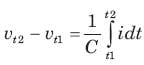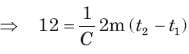12 * C  = 2 m x 10
C = 1.67 mF
Hence, Capacitance is equal to 1.67 mF.

Test: Basic Concepts - Question 17

The energy required to charge a 10 μF capacitor to 100 V is:

Detailed Solution for Test: Basic Concepts - Question 17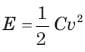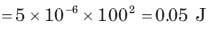Test: Basic Concepts - Question 18

The current in a 100 μF capacitor is shown in fig. If capacitor is initially uncharged, then the waveform for the voltage across it is: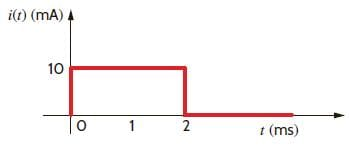Detailed Solution for Test: Basic Concepts - Question 18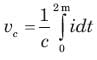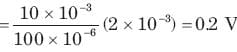This 0.2 V increases linearly from 0 to 0.2 V. Then current is zero. So capacitor hold this voltage.

Test: Basic Concepts - Question 19

The voltage across a 100 μF capacitor is shown in fig. The waveform for the current in the capacitor is: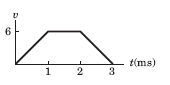Detailed Solution for Test: Basic Concepts - Question 19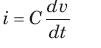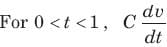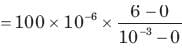= 600 mA
For 1 ms < t < 2 ms,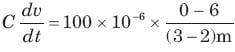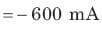Test: Basic Concepts - Question 20

The waveform for the current in a 200 μF capacitor is shown in fig. The waveform for the capacitor voltage is: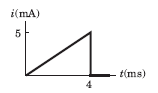Detailed Solution for Test: Basic Concepts - Question 20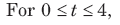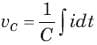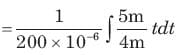= 3125 t2
At t = 4 ms, vc = 0.05 V
It will be parabolic path at t = 0
t-axis will be tangent.

## RRB JE for Electrical Engineering

2 videos|3 docs|75 tests
 Use Code STAYHOME200 and get INR 200 additional OFF Use Coupon Code
Information about Test: Basic Concepts Page
In this test you can find the Exam questions for Test: Basic Concepts solved & explained in the simplest way possible. Besides giving Questions and answers for Test: Basic Concepts, EduRev gives you an ample number of Online tests for practice

## RRB JE for Electrical Engineering

2 videos|3 docs|75 tests